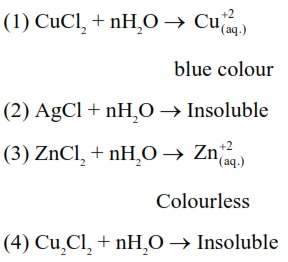# Which one of the following when dissolved in water givesQuestion:

Which one of the following when dissolved in water gives coloured solution in nitrogen atmosphere?

1. $\mathrm{CuCl}_{2}$

2. $\mathrm{AgCl}$

3. $\mathrm{ZnCl}_{2}$

4. $\mathrm{Cu}_{2} \mathrm{Cl}_{2}$

Correct Option: 1,

Solution: